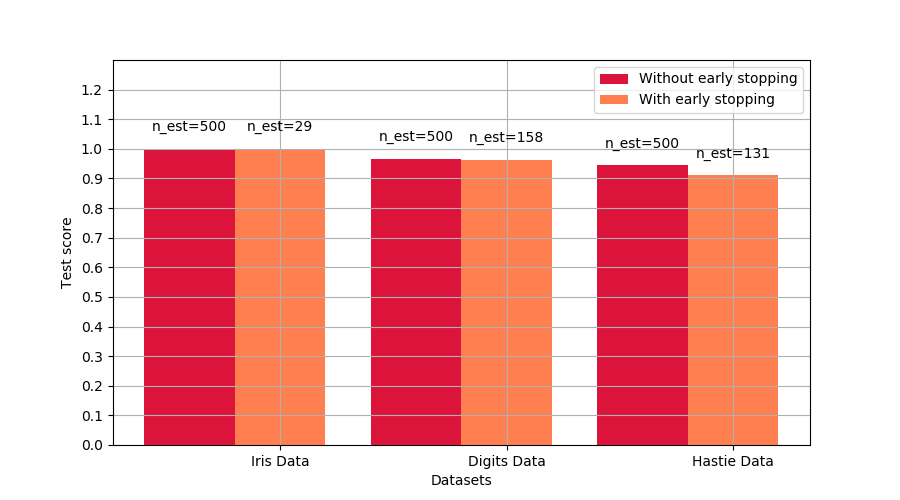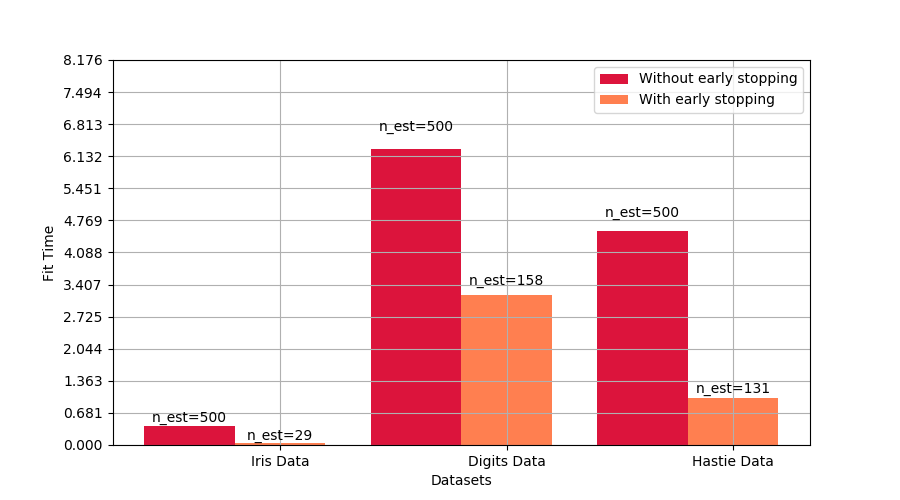# Early stopping of Gradient Boosting¶

Gradient boosting is an ensembling technique where several weak learners (regression trees) are combined to yield a powerful single model, in an iterative fashion.

Early stopping support in Gradient Boosting enables us to find the least number of iterations which is sufficient to build a model that generalizes well to unseen data.

The concept of early stopping is simple. We specify a validation_fraction which denotes the fraction of the whole dataset that will be kept aside from training to assess the validation loss of the model. The gradient boosting model is trained using the training set and evaluated using the validation set. When each additional stage of regression tree is added, the validation set is used to score the model. This is continued until the scores of the model in the last n_iter_no_change stages do not improve by atleast tol. After that the model is considered to have converged and further addition of stages is “stopped early”.

The number of stages of the final model is available at the attribute n_estimators_.

This example illustrates how the early stopping can used in the sklearn.ensemble.GradientBoostingClassifier model to achieve almost the same accuracy as compared to a model built without early stopping using many fewer estimators. This can significantly reduce training time, memory usage and prediction latency.

# Authors: Vighnesh Birodkar <vighneshbirodkar@nyu.edu>
#          Raghav RV <rvraghav93@gmail.com>

import time

import numpy as np
import matplotlib.pyplot as plt

from sklearn import ensemble
from sklearn import datasets
from sklearn.model_selection import train_test_split

print(__doc__)

data_list = [(d.data, d.target) for d in data_list]
data_list += [datasets.make_hastie_10_2()]
names = ['Iris Data', 'Digits Data', 'Hastie Data']

n_gb = []
score_gb = []
time_gb = []
n_gbes = []
score_gbes = []
time_gbes = []

n_estimators = 500

for X, y in data_list:
X_train, X_test, y_train, y_test = train_test_split(X, y, test_size=0.2,
random_state=0)

# We specify that if the scores don't improve by atleast 0.01 for the last
# 10 stages, stop fitting additional stages
validation_fraction=0.2,
n_iter_no_change=5, tol=0.01,
random_state=0)
random_state=0)
start = time.time()
gb.fit(X_train, y_train)
time_gb.append(time.time() - start)

start = time.time()
gbes.fit(X_train, y_train)
time_gbes.append(time.time() - start)

score_gb.append(gb.score(X_test, y_test))
score_gbes.append(gbes.score(X_test, y_test))

n_gb.append(gb.n_estimators_)
n_gbes.append(gbes.n_estimators_)

bar_width = 0.2
n = len(data_list)
index = np.arange(0, n * bar_width, bar_width) * 2.5
index = index[0:n]


## Compare scores with and without early stopping¶

plt.figure(figsize=(9, 5))

bar1 = plt.bar(index, score_gb, bar_width, label='Without early stopping',
color='crimson')
bar2 = plt.bar(index + bar_width, score_gbes, bar_width,
label='With early stopping', color='coral')

plt.xticks(index + bar_width, names)
plt.yticks(np.arange(0, 1.3, 0.1))

def autolabel(rects, n_estimators):
"""
Attach a text label above each bar displaying n_estimators of each model
"""
for i, rect in enumerate(rects):
plt.text(rect.get_x() + rect.get_width() / 2.,
1.05 * rect.get_height(), 'n_est=%d' % n_estimators[i],
ha='center', va='bottom')

autolabel(bar1, n_gb)
autolabel(bar2, n_gbes)

plt.ylim([0, 1.3])
plt.legend(loc='best')
plt.grid(True)

plt.xlabel('Datasets')
plt.ylabel('Test score')

plt.show()## Compare fit times with and without early stopping¶

plt.figure(figsize=(9, 5))

bar1 = plt.bar(index, time_gb, bar_width, label='Without early stopping',
color='crimson')
bar2 = plt.bar(index + bar_width, time_gbes, bar_width,
label='With early stopping', color='coral')

max_y = np.amax(np.maximum(time_gb, time_gbes))

plt.xticks(index + bar_width, names)
plt.yticks(np.linspace(0, 1.3 * max_y, 13))

autolabel(bar1, n_gb)
autolabel(bar2, n_gbes)

plt.ylim([0, 1.3 * max_y])
plt.legend(loc='best')
plt.grid(True)

plt.xlabel('Datasets')
plt.ylabel('Fit Time')

plt.show()Total running time of the script: ( 0 minutes 15.622 seconds)

Gallery generated by Sphinx-Gallery